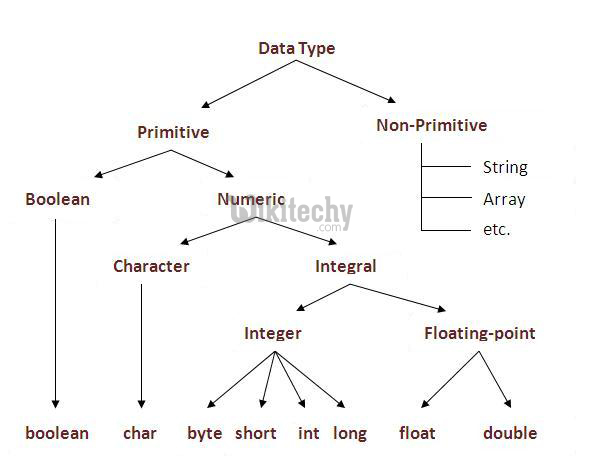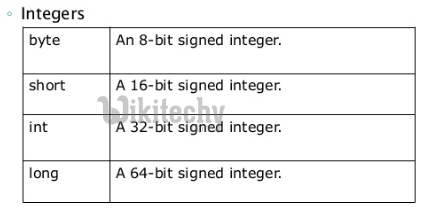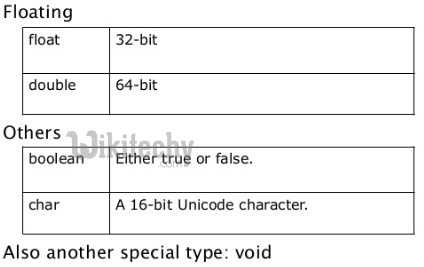# java tutorial - Java datatypes and literals | Primitive and reference data types, literals - java programming - learn java - java basics - java for beginners

• Variables are nothing more than reserved memory locations for storing values. This implies when you create variable, you reserve a few space in memory.
• Based on the type of data which is assigned to the variable, the OS allocates memory and chose what should be stored in the reserved memory.
• Hence, by assigning several data types to variables, the programming language Java will store integers, decimals and characters in these variables.

There are two types of data in Java:

• Simple or primitive data types;
• Referential data types (reference / object).## Primitive data types

• There are 8 types of data are supported by Java. The most important data types are predefined by the language and are named for the keyword.
• Now let's look in detail at these eight basic data types existing in the Java programming language.## Byte type in java

• The byte data type is an 8-bit signed integer.
• The minimum value is -128 (-2 7).
• The maximum value is 127 (inclusive) (2 7 -1).
• The default is 0.
• byte is designed to save space in large arrays, mostly instead of integers, because byte is four times smaller than int.

Example:

## Short type in Java

• The short data type is a 16-bit signed integer.
• The minimum value is -32768 (-2 15).
• The maximum value is 32,767 (inclusive) (2 15 -1).
• The short type in Java can also be used to save memory as byte. The size of short is 2 times less than int.
• The default is 0.
• Example:

## Int type in java

• In Java, the int data type is a 32-bit signed integer.
• The minimum size int is 2 147 483 648 (-2 31).
• The maximum value is 2,147,483,647 (inclusive) (2 31 -1).
• The int type is usually used for integer values. If there is no concern about memory.
• The default is 0.
• Example:

## long type in Java

• The long data type is a 64-bit signed integer.
• The minimum value is - 9,223,372,036,854,775,808 (-2 63 ).
• The maximum value is 9,223,372,036,854,775.807 (inclusive). (2 63 -1).
• In Java Applies when a wider range than int is required.
• The default is 0L.
• Example:

## Float type in java

• The data type float is a single precision 32-bit IEEE 754 floating point.
• The float type is mainly used to store memory in large arrays of floating-point numbers.
• The default is 0.0f.
• The float type should never be used for an exact value, such as currency.
• Example:

## double datatype in java

• The double data type is a double-precision 64-bit IEEE 754 floating point.
• Usually used for decimal values.
• The double type should never be used for an exact value, such as a currency.
• The default is 0.0d.
• Example:

## Boolean datatype in java

• The boolean data type is one bit of information.
• There are only two possible values: true and false.
• It is intended for simple signs that allow you to track conditions true or false.
• The default is false.
• Example:

## char datatype in java

• The char data type is one 16-bit Unicode character.
• The minimum value is "\ u0000" (or 0).
• The maximum value is "\ uffff" (or 65535 inclusive).
• In Java, a char is needed to store any character.
• Example:

## Reference data types

• Reference variables are created using certain class constructors. They are designed to access objects. These variables are declared with a specific type that can not be changed. For example, Employee, Puppy, etc.
• Class objects and different kinds of array variables fall under the reference data type .
• In Java, by default, the value of several reference variable is invalid (null).
• A reference variable can be used to refer several object declared or of any compatible type.
• Example:

## Literals in Java

Literals in Java represent the representation of the source code as a fixed value. A Literals is represented directly in the code with no computation. A literal in Java can be assigned to several variables from the core type. For example:

• Byte, int, long, and short can be expressed in decimal (base 10), hexadecimal (base 16), or octal (base 8) by the calculus.
• When using literals in Java, the 0 prefix is used to specify the octal system, and the 0x prefix indicates a hexadecimal system. For example:
• String literals in the Java language are defined as in most other languages, enclosing a sequence of characters between a pair of double quotes. Examples of string literals:

Types of literals String and char can contain any Unicode characters. For example:

The Java language supports several special escape sequences for the String and char literals:

 Notation Performance \ n New line (0x0a) \ r Carriage return (0x0d) \ f Page run (0x0c) \ b Return to step (0x08) \ s space (0x20) \ t Tabulation \ " Double quote \ ' Apostrophe \\ Backslash \ ddd Octal symbol (ddd) \ uxxxx Hexadecimal character UNICODE (xxxx)